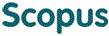### BROWSE

7 363

Cited 0 times inStraght full archwire에 의하여 발생하는 force system에 관한 분석

Other Titles
Analysis of force system developed by straight full archwire
Authors
최광철
Issue Date
1992
Description
치의학과/석사
Abstract
[한글] Edgewise bracket에 unbent straight arch wire를 장착했을 때 각 bracket에 발생하는force system은 statically indeterminate system이기 때문에 임상적으로 측정이 어렵다. 이에 본 연구는 linear beam theory를 도입하여 full arch에 straight wire가 장착되어있는 경우 geometry(a/b), 재질, 단면의 모양, interbracket distance에 관계없이 발생 가능한 모든 상태를 설명하고 다음과 같은 결과를 얻었다. 1. Bilateral fixed beam의 양끝에서 발생하는 force system을 알 수 있는 계산식을 얻었다. 2. Bracket의 moment to force ratio는 일정한 geometry(a/b)에서 interbracket distance에 대한 일정한 비율을 가지며 elastic range내에서 wire의 변형정도에 영향을 받지 않는다. 3. Full arch wire 장착시 wire의 영구변형의 발생여부와 발생위치를 예측할 수 있다. ANALYSIS OF FORCE SYSTEM DEVELOPED BY STRAIGHT FULL ARCHWIRE Kwang-Chul Choy, D.D.S. Dept. of Orthodontics, College of Dentistry, Yonsei University (Directed by Prof. Byung Wha Sohn, D.D.S., M.S.D., Ph.D.) Determination of force system and prediction of side effects from unbent straight wire engaged in edgewise bracket is clinically very difficult because it is statically indeterminate system. This study is to develop a linear beam theory that explains the force system for straight wire engaged in edgewise bracket regardless of geonletry(a/b), material, cross section of wire and interbracket distance. The results were as follows; 1. The formula was derived to explain the force system for bilateral fixed beam 2. In two bracket system under the constant geometry(a/b) the moment to force ratio had a constant ratio to the interbracket distance regardless of amount of deflection within the elastic range. 3. The possibility and location of permanent deformation can be predicted.
[영문] Determination of force system and prediction of side effects from unbent straight wire engaged in edgewise bracket is clinically very difficult because it is statically indeterminate system. This study is to develop a linear beam theory that explains the force system for straight wire engaged in edgewise bracket regardless of geonletry(a/b), material, cross section of wire and interbracket distance. The results were as follows; 1. The formula was derived to explain the force system for bilateral fixed beam 2. In two bracket system under the constant geometry(a/b) the moment to force ratio had a constant ratio to the interbracket distance regardless of amount of deflection within the elastic range. 3. The possibility and location of permanent deformation can be predicted.
Full Text
https://ymlib.yonsei.ac.kr/catalog/search/book-detail/?cid=CAT000000003755
Files in This Item:
제한공개 원문입니다.
Appears in Collections:
2. College of Dentistry (치과대학) > Dept. of Advanced General Dentistry (통합치의학과) > 2. Thesis
URI
https://ir.ymlib.yonsei.ac.kr/handle/22282913/117144
사서에게 알리기